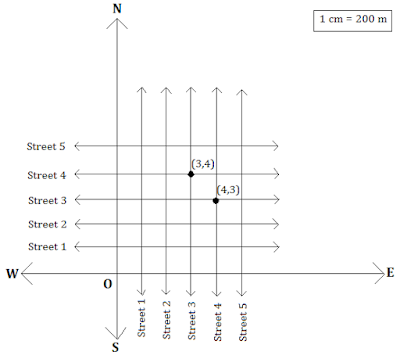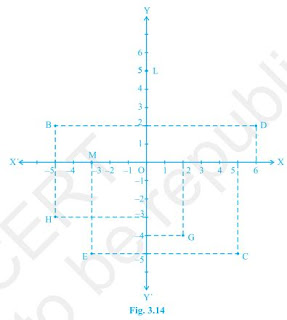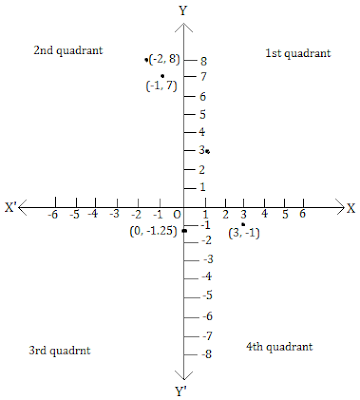# NCERT Solutions for Class 9th: Ch 3 Coordinate Geometry Maths

#### NCERT Solutions for Class 9th: Ch 3 Coordinate Geometry Maths

Page No: 53

Exercise 3.1

1. How will you describe the position of a table lamp on your study table to another person?

To describe the position of a table lamp on the study table, we have two take two lines, a perpendicular and horizontal. Considering the table as a plane and taking perpendicular line as Y axis and horizontal as X axis. Take one corner of table as origin where both X and Y axes intersect each other. Now, the length of table is Y axis and breadth is X axis. From The origin, join the line to the lamp and mark a point. Calculate the distance of this point from both X and Y axes and then write it in terms of coordinates.
Let the distance of point from X axis is x and from Y axis is y then the the position of the table lamp in terms of coordinates is (x,y).

2. (Street Plan) : A city has two main roads which cross each other at the centre of the city. These two roads are along the North-South direction and East-West direction.
All the other streets of the city run parallel to these roads and are 200 m apart. There are 5 streets in each direction. Using 1cm = 200 m, draw a model of the city on your notebook. Represent the roads/streets by single lines. There are many cross- streets in your model. A particular cross-street is made by two streets, one running in the North - South direction and another in the East - West direction. Each cross street is referred to in the following manner : If the 2nd street running in the North - South direction and 5 th in the East - West direction meet at some crossing, then we will call this cross-street (2, 5). Using this convention, find:
(i) how many cross - streets can be referred to as (4, 3).
(ii) how many cross - streets can be referred to as (3, 4)(i) Only one street can be referred to as (4, 3) as we see from the figure.
(ii) Only one street can be referred to as (3, 4) as we see from the figure.

Page No: 60

Exercise 3.2

1. Write the answer of each of the following questions:
(i) What is the name of horizontal and the vertical lines drawn to determine the position of any point in the Cartesian plane?
(ii) What is the name of each part of the plane formed by these two lines?
(iii) Write the name of the point where these two lines intersect.

(i) The name of horizontal lines and vertical lines drawn to determine the position of any point in the Cartesian plane is x-axis and y-axis respectively.
(ii) The name of each part of the plane formed by these two lines x-axis and y-axis is quadrants.
(iii) The point where these two lines intersect is called origin.

2. See Fig.3.14, and write the following:
(i) The coordinates of B.
(ii) The coordinates of C.
(iii) The point identified by the coordinates (-3, -5).
(iv) The point identified by the coordinates (2, -4).
(v) The abscissa of the point D.
(vi) The ordinate of the point H.
(vii)The coordinates of the point L.
(viii) The coordinates of the point M.(i) The coordinates of B is (-5, 2).

(ii) The coordinates of C is (5, -5).

(iii) The point identified by the coordinates (-3, -5) is E.

(iv) The point identified by the coordinates (2, -4) is G.

(v) Abscissa means x coordinate of point D. So, abscissa of the point D is 6.

(vi) Ordinate means y coordinate of point H. So, ordinate of point H is -3.

(vii) The coordinates of the point L is (0, 5).

(viii) The coordinates of the point M is (- 3, 0).

Page No: 65

Exercise 3.3

1. In which quadrant or on which axis do each of the points (-2, 4), (3, -1), (-1, 0), (1, 2) and (-3, -5) lie? Verify your answer by locating them on the Cartesian plane.